International
Tables for
Crystallography
Volume B
Reciprocal space
Edited by U. Shmueli

International Tables for Crystallography (2010). Vol. B, ch. 4.1, pp. 488-489   | 1 | 2 |

Section 4.1.4. Scattering of neutrons by thermal vibrations

B. T. M. Willisa*

aDepartment of Chemistry, Chemistry Research Laboratory, University of Oxford, Mansfield Road, Oxford OX1 3TA, England
Correspondence e-mail: bertram.willis@chem.ox.ac.uk

4.1.4. Scattering of neutrons by thermal vibrations

| top | pdf |

The amplitude of the X-ray beam scattered by a single atom is denoted by the atomic scattering factor f(Q). The corresponding quantity in neutron scattering is the scattering amplitude or scattering length b of an atom. b is independent of scattering angle and is also independent of neutron wavelength apart from for a few isotopes (e.g. 113Cd, 149Sm) with resonances in the thermal neutron region.

The scattering amplitude for atoms of the same chemical element can vary randomly from one atom to the next, as different amplitudes are associated with different isotopes. If the nucleus has a nonzero spin, even a single isotope has two different amplitudes, dependent on whether the nuclear spin is parallel or antiparallel to the spin of the incident neutron. If there is a variation in the amplitude associated with a particular type of atom, some of the waves scattered by the atoms will interfere with one another and some will not. The first part is called coherent scattering and the second incoherent scattering. The amplitude of the coherent scattering is determined by the mean atomic scattering amplitude, averaged over the various isotopes and spin states of the atom, and is known as the coherent scattering length,.

A crucial difference between neutrons and X-rays concerns their energies: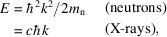where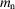is the neutron mass and c the velocity of light. AtÅ, neutrons have an energy of 0.08 eV or a temperature of about 800 K; for X-rays of this wavelength the corresponding temperature exceeds 108 K! Thermal neutrons have energies comparable with phonon energies, and so inelastic scattering processes, involving the exchange of energy between neutrons and phonons, produce appreciable changes in neutron energy. (These changes are readily determined from the change in wavelength or velocity of the scattered neutrons.) It is customary to refer to the thermal diffuse scattering of neutrons as inelastic neutron scattering' to draw attention to this energy change.

For a scattering process in which energy is exchanged with just one phonon, energy conservation giveswhere E0 and E are the energies of the neutron before and after scattering and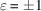. Ifthe neutron loses energy by creating a phonon (phonon emission'), and ifit gains energy by annihilating a phonon (`phonon absorption').

The partial differential scattering cross sectiongives the probability that neutrons will be scattered into a small solid angleabout the direction k with a change of energy betweenand. This cross section can be split into two terms, known as the coherent and incoherent cross sections: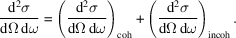The coherent cross section depends on the correlation between the positions of all the atoms at different times, and so gives interference effects. The incoherent cross section depends only on the correlation between the positions of the same atom at different times, giving no interference effects. Incoherent inelastic scattering is the basis of a powerful technique for studying the dynamics of molecular crystals containing hydrogen (Boutin & Yip, 1968).

The coherent scattering cross sectioncan be expanded, as in the X-ray case [equation (4.1.3.2)], into terms representing the contributions from zero-phonon, one-phonon, two-phonon … scattering. To determine phonon dispersion relations, we measure the one-phonon contribution and this arises from both phonon emission and phonon absorption: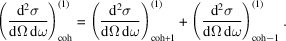The superscript (1) denotes a one-phonon process, and the subscript +1 (−1) indicates emission (absorption).

The emission cross section is given by (Squires, 1978)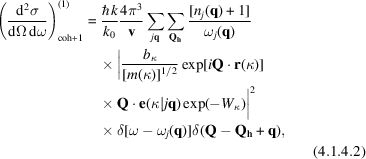whereas for phonon absorption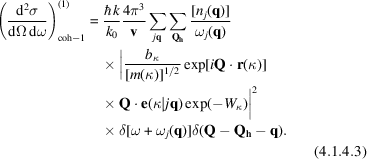The first delta function in these two expressions embodies energy conservation,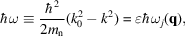and the second embodies conservation of momentum,The phonon population number,, tends to zero as[see equation (4.1.2.6)], so that the one-phonon absorption cross section is very small at low temperatures where there are few phonons for the radiation to absorb. Comparison of equations (4.1.4.2) and (4.1.4.3) shows that there is always a greater probability that the neutrons are scattered with energy loss rather than with energy gain.

Normally it is not possible in X-ray experiments to distinguish between phonon emission and phonon absorption, and the measured cross section is obtained by summing over all energy transfers. The cross section for X-rays can be derived from the neutron formulae, equations (4.1.4.2) and (4.1.4.3), by puttingand by replacingwith. Integration overand addition of the parts for emission and absorption gives the X-ray formula (4.1.3.6).

The theory of neutron scattering can also be formulated in terms of thermal averages known as Van Hove correlation functions (Van Hove, 1954). For example, the partial differential cross section for coherent scattering is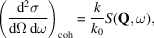where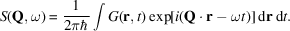is the Fourier transform in space and time of, the time-dependent pair-correlation function. The classical interpretation ofis that it is the probability of finding any atom at time t in a volume, if there is an atom at the origin at time zero.

References

Boutin, H. & Yip, S. (1968). Molecular Spectroscopy with Neutrons. Cambridge: MIT Press.
Squires, G. L. (1978). Introduction to the Theory of Thermal Neutron Scattering. Cambridge University Press.
Van Hove, L. (1954). Correlations in space and time and Born approximation scattering in systems of interacting particles. Phys. Rev. 95, 249–262.